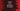# Sort a python dictionary by values## Dictionary sorting in Python :

Dictionary is used to store key-value pairs. We can sort the items in a dictionary based on the values. In this tutorial, I will show you how to sort a python dictionary based on its values.

## Using sorted with operator module :

We can use sorted function with operator module to sort all dictionary values in python. Operator module has one function called itemgetter() that can be used to compare the dictionary items based on its values :

``````import operator

dict = {'one': 1,'four': 4,'five': 5, 'two': 2,'three': 3}
print(dict)

sorted_dict = sorted(dict.items(), key=operator.itemgetter(1))
print(sorted_dict)``````

### Output :

``````{'one': 1, 'four': 4, 'five': 5, 'two': 2, 'three': 3}
[('one', 1), ('two', 2), ('three', 3), ('four', 4), ('five', 5)]``````

The output is not a dictionary. It is a list of tuples sorted by the values of each element. Each tuple contains the key and values of the dictionary.

## Using sorted, lambda function :

We can also use one lambda function instead of using the operator module :

``````dict = {'one': 1,'four': 4,'five': 5, 'two': 2,'three': 3}
print(dict)

sorted_dict = sorted(dict.items(), key=lambda x: x)
print(sorted_dict)``````

### Output :

``````{'one': 1, 'four': 4, 'five': 5, 'two': 2, 'three': 3}
[('one', 1), ('two', 2), ('three', 3), ('four', 4), ('five', 5)]``````

## Using list comprehension :

We can use list comprehension with the sorted function :

``````dict = {'one': 1,'four': 4,'five': 5, 'two': 2,'three': 3}
print(dict)

sorted_dict = sorted((value, key) for (key,value) in dict.items())
print(sorted_dict)``````

It will create one list of tuples with (value, key) items.

``````{'one': 1, 'four': 4, 'five': 5, 'two': 2, 'three': 3}
[(1, 'one'), (2, 'two'), (3, 'three'), (4, 'four'), (5, 'five')]``````